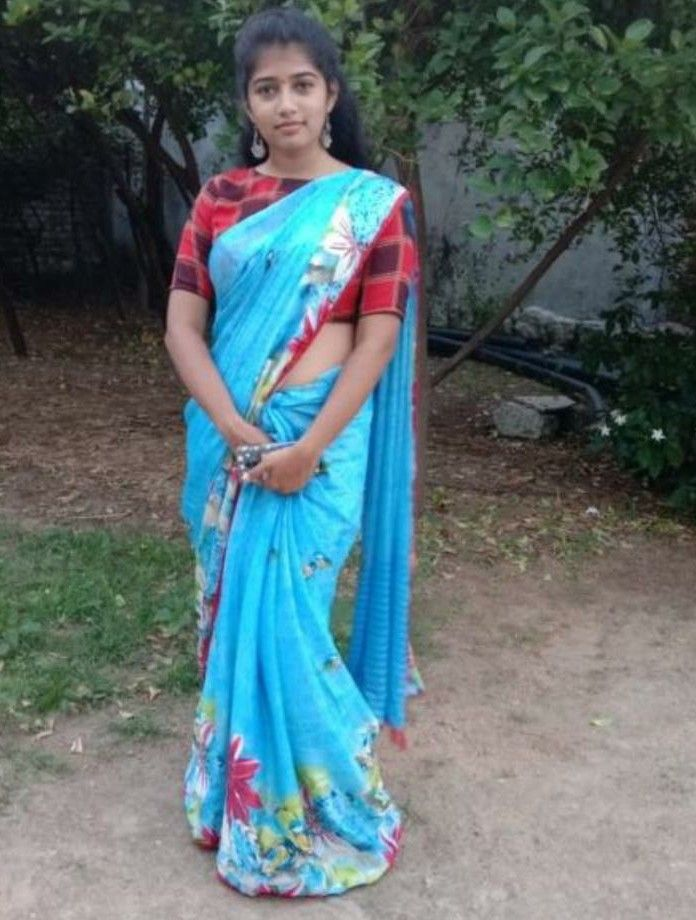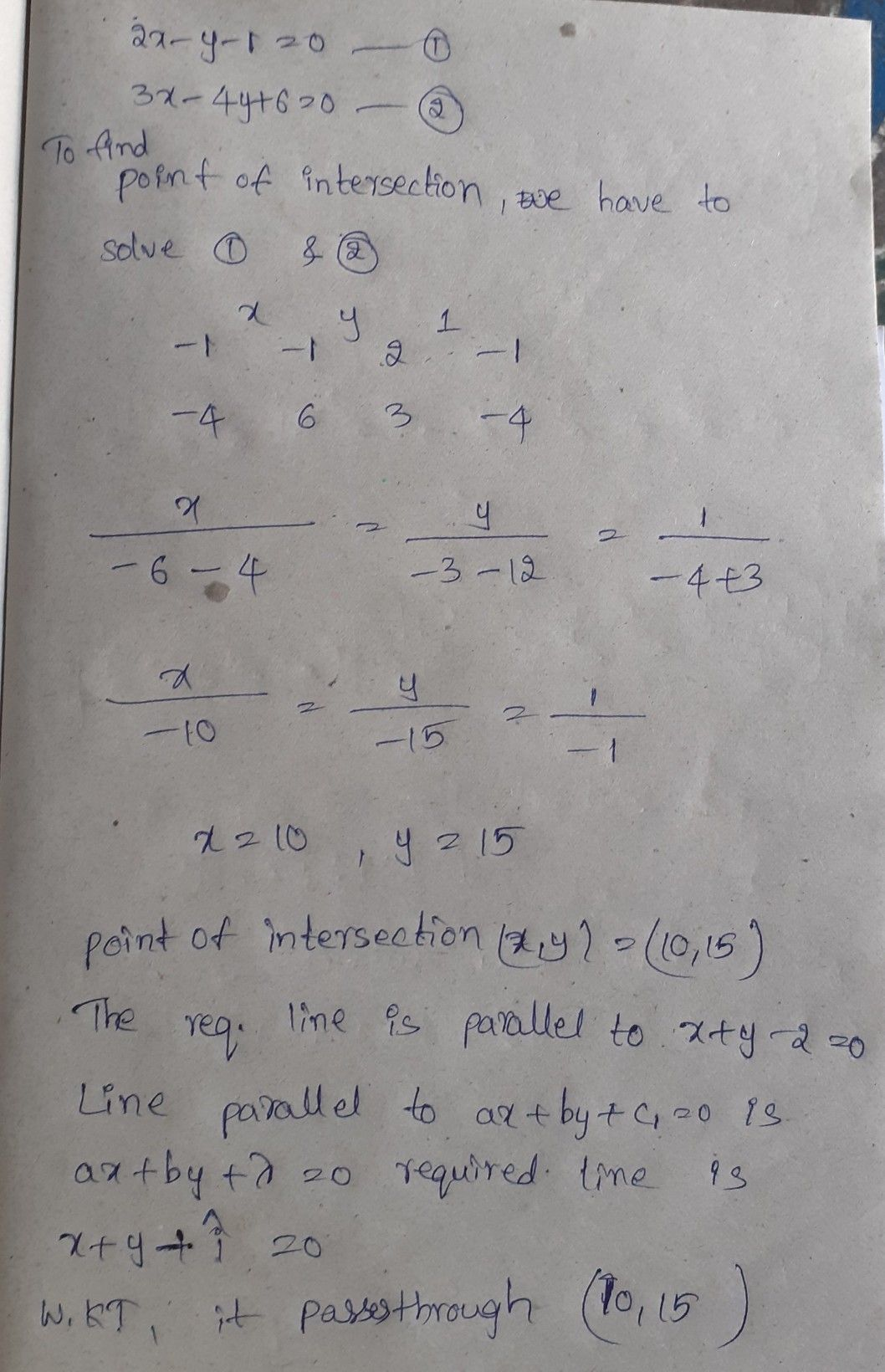Symbol
Problem7. $\left(a\right)$ Find the equation of the lines which is parallell to the line $3x+4y+7=0$ and is at a distance 2 from it. $\left(b\right)$ Find the equations of the $diagona|$ of the parallelogram formed by the lines $ax+by=0$ $ax+$ + $by+c=0.x+my=0$ and $dlx+my+n=0$ What is the condition that this will be a rhombus ? (c) Find the equation of the line passing through the intersection $of2x-y-1=0$ and $3x-$ $4y+6=0$ and parallel to the line $cx+y-2=0$ $\left(d\right)$ Find the equation ofthe line passing through the point of intersection of lines.x+3y+2%D $0andx-2y-4=0a$ and perpendicular to the line $2y+5x-9=0$ $\left(c\right)$ Find the equation of the line passing through intersection of the lines.x+3y-1=0 and 3x- $y+1=0$ $4\right)$ and the centroid of the triangle whose vertices are the points $\left(3,-1\right).\left(1.3\right)$ and (2,
1st-6th grade
Other
Search count: 109
SolutionQanda teacher - alekya